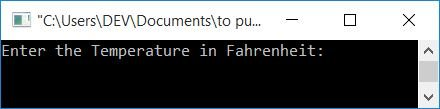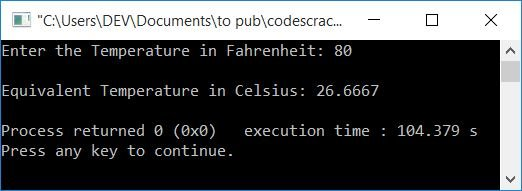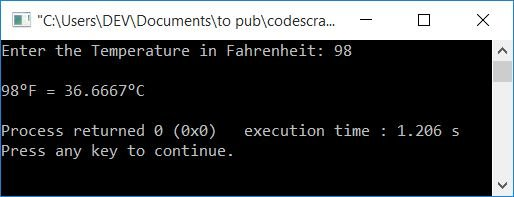# C++ program to convert Fahrenheit to Celsius

In this article, you will learn and get code on temperature conversion, that is, the conversion of a temperature from Fahrenheit to Celsius (or Centigrade) with and without using a function in C++.

### Fahrenheit to Celsius formula

To convert a temperature given in Fahrenheit to its equivalent value in Celsius, use the following formula:

`C = (F-32)*(100/180)`

where C and F indicate the values of Fahrenheit and Celsius, respectively. This formula can also be written as:

`C = (F-32)/(180/100)`

Or,

`C = (F-32)/1.8`

If you're curious to know about the concept behind the formula, then refer to The Celsius to Fahrenheit Formula Explained.

## Fahrenheit to Celsius in C++

To convert temperature from Fahrenheit to Celsius in C++ programming, you have to ask the user to enter the temperature in Fahrenheit first. and then convert it into its equivalent value in Celsius with the formula given above.

The question is, "Write a program in C++ that receives temperature in degrees Fahrenheit and prints its equivalent value in degrees Celsius." Here is its answer:

```#include<iostream>
using namespace std;
int main()
{
float fahrenheit, celsius;
cout<<"Enter the Temperature in Fahrenheit: ";
cin>>fahrenheit;
celsius = (fahrenheit-32)/1.8;
cout<<"\nEquivalent Temperature in Celsius: "<<celsius;
cout<<endl;
return 0;
}```

This program was built and runs under the Code::Blocks IDE. Here is its sample run:Now supply the temperature input in Fahrenheit, say 80, and press the ENTER key to convert, which prints its equivalent value in Celsius as shown in the snapshot given below:### Fahrenheit to Celsius conversion in C++, using a user-defined function

Let's create the same-purpose program using a user-defined function, FahrenheitToCelsius(). This function takes a Fahrenheit value as its argument and returns its equivalent Celsius value. Its return value gets initialized to Celsius inside the main() function. So print the value of Celsius.

```#include<iostream>
using namespace std;
float FahrenheitToCelsius(float);
int main()
{
float fahrenheit, celsius;
cout<<"Enter the Temperature in Fahrenheit: ";
cin>>fahrenheit;
celsius = FahrenheitToCelsius(fahrenheit);
cout<<endl<<fahrenheit<<"\370F = "<<celsius<<"\370C";
cout<<endl;
return 0;
}
float FahrenheitToCelsius(float f)
{
float c;
c = (f-32)/1.8;
return c;
}```

Here is its sample run with user input as 98:Note: The \370 is used to print degree (o).

#### The same program in different languages

C++ Quiz

« Previous Program Next Program »